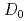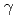Nuclear reaction calculations using the statistical model require information of the nuclear level density. While the phenomenological models such as Fermi Gas model are often used to calculate the nuclear level density in usual nuclear data evaluations, reliability of such level density depends on parameter adjustments using experimental values. Therefore, development of microscopic calculation methods that does not depend on parameter adjustments is desired. One of the microscopic methods to calculate the nuclear level density is the combinatorial method that uses single-particle levels obtained from Hartree-Fock theory, and its reliability has been discussed through the reproducibility of the s-wave neutron resonance spacing. However, comparisons of cross sections with experiments are only preformed for (n,) reaction of some nuclei, and is still insufficient. Therefore, in this work, we calculate the nuclear level density using the combinatorial method, and apply it to the nuclear reaction calculations such as (n,) reaction and (n,2n) reaction calculations. The cross sections of these reactions adding to the s-wave neutron resonance spacingfor stable nuclei are compared with the experiments systematically, and then the reliability of the nuclear level density is discussed.